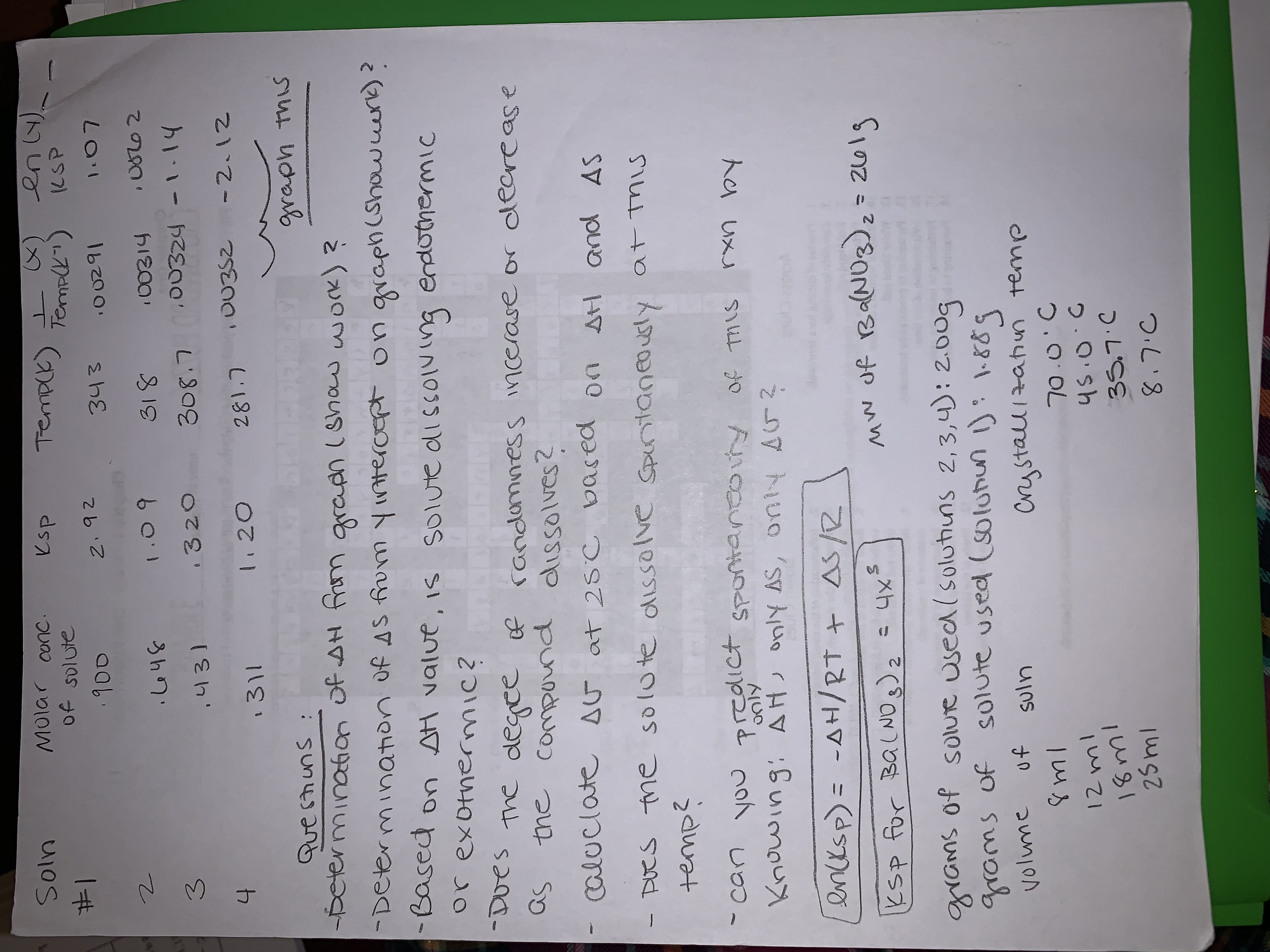# looking for a tutor to help answer the delta g questions in the image attached below (last 3 dashes). 4. Calculate ΔG at 25°C based on ΔH and ΔS 5. does the solute dissolve spontaneously at this temp? 6. Can you predict spontaneity of this reaction by knwing only ΔH, ΔS, and ΔG? I have labeled the questions that need to be answered as "questions" and the information below the section labeled "questions" is information that can be useful in helping to answer the 3 questions i indicated. for the calculation of delta g, delta s is 133.3j/molk and delta h is 42857j/mol.